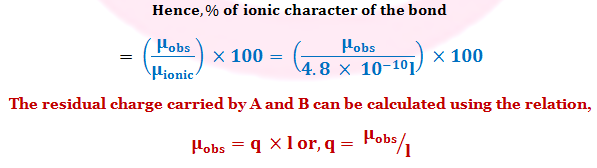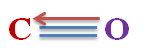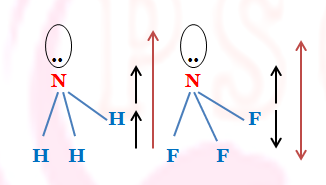## Articles by "INORGANIC CHEMISTRY"

Showing posts with label INORGANIC CHEMISTRY. Show all posts

## Application of Dipole Moment in Chemistry:

Different applications are taken one after another.

### 1.Determination of Partial Ionic Character and Residual Charge on the Constituent Atoms in a Molecule:

Let us consider a molecule A - B having the dipole moment μobs and the bond length l cm. if the shared electron pair lies at the midpoint of the atoms, the bond will be purely covalent and the present of ionic character is zero.
But if the bond is 100% ionic, and B is more electronegative then A, B will carry unit negative charge and A uni-positive charged respectively. In that case the dipole moment of the molecule would be,
 μionic = e × ℓ = (4.8 × 10⁻¹⁰) ℓ esu cm
But the dipole moment of AB is neither zero or nor μionic .Determination of Partial Ionic Character and Residual Charge
The displacement of HCl is 1.03 Debye and its bond length is 1.27 A.
calculate the (a) charge on the constituent atom and
(b) the % of the ionic Character of HCl.
 Given, μobs = 1.03 Debye  = 1.03 × 10⁻¹⁸ esu cm and length ℓ  = 1.27 × 10 ⁻⁸ cm.
(a). Hence the charge on the constituent atom(q),
 = μobs/ℓ = (1.03 × 10⁻¹⁸ )/( 1.27 × 10⁻⁸) = 0.8 × 10⁻¹⁰ esu
(b) The % of the ionic Character of the molecule,
 (μobs/μionic ) × 100 =(1.03×10⁻¹⁸)/(4.8×10⁻¹⁰×1.27×10⁻⁸)×100  = 16.89%

### Determination of molecular radius from induced polarization (Pi) :

 The induced polarization (Pi) = (4/3) π N0 αi
But when the substance is in the gaseous state,
 Pi = {(D0-1)/(D0+2)} M/ρ  (for the covalent substance)
The value of D൦ is close to unity under this condition,
 Hence, {(D0 - 1)/3} × 22400  = ( 4/3 ) π N0 r³
At NTP, M/ρ = molar volume = 22400cc/mole and αi= r³ taking the spherical shape of the molecule.
 or, r³ = (22400/4πN0 ) (D0 - 1)  = 2.94 ×10⁻²¹ (D൦ - 1)
Hence, radius of the molecule r can be determined by measuring D൦ of the substance at NTP.

### Determination of molecular structure :

#### (i) Mono-atomic Molecules (A) :

The mono-atomic inert gases are non-polar and it indicates the symmetrical charge distribution in molecule.

#### (ii) Di-atomic Molecules (AX) :

The homonucler diatomic molecules are largely non-polar such as N₂, O₂, Cl₂ indicating that there are symmetrical charge distributions. The bonding electron pair is equally shared by the two bonding atoms. However, Br₂, I₂ have non zero vale of dipole moment and this indicates the unsymmetrical charge distribution,
 I+ ➖ I-
 Compounds Dipole Moment Structure
 H2 0 H — H Cl2 0 Cl ― Cl
Heteronucler diatomic molecules are always polar due to difference of electronegativity of the constituent atoms. The example are , HCl, HBr, HF etc. this indicates that electron pair is not equally shared and shifted to the more electronegative atom.
 δ+ → δ- HCl 1.03 D H ― Cl
 δ+ → δ- HBr 0.79 D H ― Br
 δ+ → δ- HI 0.38 D H ― I
 δ+ → δ- HF 2.00 D H ― F
The Difference between electronegativity of Carbon and Oxygen Is large but the dipole moments of carbon monoxide is very low - Why?
However, in CO, there are large difference of electronegativity between C and O but the molecule is very low value of dipole moment. This suggested that the charge density in O-atom is somehow back-donated to C-atom. This explain by forming a coordinate covalent bond directing towards C-atom.Formation of Coordinate Covalent Bond in Carbon Monoxide

### (iii) Tri-atomic Molecules (AX₂):

The molecules, like CO₂, BeCl₂, BeF₂, SnCl₂ etc have zero dipole moment indicating that the molecules have symmetrical linear structure.
For example, CO₂ has structure, The electric moment of one C - O bond ( known as bond moment ) cancel the electric moment of the other C - O bond.
 δ- ← δ+ → δ- O = C = O
 δ- ← δ+ → δ- Cl = Be = Cl
The electric moment associated with the bond arising from difference of electronegativity is called the bond moment(m). In molecules, the vectorial addition of the bond moments (m) gives the resultant dipole moment(μ) of the molecule.
 μ² = m12 + m22 + 2m1m2Cosθ
Where, m1 and m2 are the bond moments.
These helps to calculate the bond angle (θ) in the molecule. Thus in the carbon dioxide molecule, μ = 0 and m1 = m2
hence, 0 = 2m²(1 + cosθ)
or, θ = 180°
that is the molecule is linear.
For Non-Linear Structure
Another type of molecules such as, H₂O, H₂S, SO₂᠌᠌ etc. have μ ≠ 0 indicating that they have non linear structure. The bond angle can be calculated from the bond moments of the molecules.
H₂O molecule has a dipole moment-Explain. Does it invalidate a linear structure ?Dipole Moment of H₂O and H₂S
 For Water (H₂O) , μ = 1.84 D  and mOH = 1.60 D  Thus, μ² = 2 m² (1+ cosθ )  or, (1.84)² = 2 (1.60)² (1+ cosθ )  or, θ = 105°
The contribution of non-bonding electrons towards the total dipole moment is included within the bond moment.
The bond angle in H₂S is 97° and dipole moment = 0.95 D . Find the bond moment of the S - H link. ( Given, Cos97° = -0.122)
 We have, μ = 0.95 D and θ = 97° .  From the equation,  μ² = 2 m² (1+ cosθ )  Putting the value we have,  (0.95)² = 2 m² (1 + cos97° )  here m = mS-H  or, 0.9025 = 2 m² (1-0.122)  or, m² = 0.9025/ (2 × 0.878)  or, m² = 0.5139  or, m = 0.72
Thus the bond moment of the S - H link is 0.72 D

#### (iv) Tetra Atomic Molecules (AX₃) :

The molecules like BCl₃, BF₃ etc have dipole moment zero indicating that they have regular planar structure. There halogen atoms are on a plane at the corner of the equilateral triangle and B atom is at the intersection of the molecules.Regular Planar Structure Of BF₃
Show that the bond moment vectors of BF₃ molecule adds up to zero.Resultant Dipole Moment of BF₃
While other type of the molecule such as NH₃, PH₃, AsH₃ are polar (μ≠0) indicating that that the molecule have a pyramidal structure in which the three H-atoms are on the plane and N-atom at the apex of the pyramid in NH₃.
But NF₃ has very small dipole moment though there is great difference of electronegativity between N and F atoms and similar structure of NH₃.Resultant Dipole Moment of NH₃ and BF₃
Low value of μ of NF₃ is explain by the fact that resultant bond moment of the three N - F bonds is acting in opposite direction to that of the lone pair placed at the N-atom. But in NH₃, the resultant bond moment is acting in the same direction as that of the lone pair electrons.

## Method of Balancing Oxidation - Reduction Reactions:

Oxidation and reduction are always found to go hand in hand during a redox reaction. Whenever an element or compound is oxidized, another element or another compound must be reduced. An oxidant is reduced and simultaneously the reductant is oxidized.It follows from our above discussion that we should be able to balance oxidation reduction reactions on the basis of
(1) Ion - Electron Method of Balancing Oxidation - Reduction Reactions.
(2) Oxidation Number Method of Balancing Oxidation - Reduction Reactions.

### 1. Ion - Electron Method of Balancing Oxidation - Reduction Reactions:

(i) Ascertain the Reactants and Products, and their chemical formulas.
(ii) Set up the partial equations representing the reduction of the oxidant, and the oxidation of the reductant.
(iii) If the reaction occurs in acid medium use requisite number of H⁺ for balancing the number of atoms involved in the partial equation. for alkaline medium use OH⁻ ions.
(iv) Balancing the charge in the partial equations by adding suitable number of electrons. These electrons indicate the electrons involves in the oxidation or the reduction half reactions.
(v) Multiply each partial equation by a suitable factor so each of the two partial equations involves the same number of electrons.
(vi) Add the partial equations and cancel out species which appear on both sides of the equations.
Some examples will now needed to elucidate the rules.
 In aqueous acid medium potassium permanganate oxidizes a ferrous ion to the ferric state.
In this reaction permanganate ion is the oxidant and ferrous ion the reductant.
The left hand side of the ultimate equation will carry, MnO₄⁻ (KMnO₄), H⁺ (H₂SO₄) and Fe²⁺ (FeSO₄).
The Right hand side will have as products, Mn²⁺ (MnSO₄), H₂O and Fe³⁺ [Fe₂(SO₄)₃].
The partial equation representing the reduction of the oxidant MnO₄⁻ will involve,
MnO₄⁻ Mn²⁺
Since the reaction occurs in acid medium we utilize eight H⁺ ions to balance the four oxygen atoms in MnO₄⁻.
Thus,  MnO₄⁻+8H⁺ → Mn²⁺+4H₂O
Since the above partial equation is still unbalanced from the viewpoint of charge, the equa-tion is balanced by bringing in five electrons:
 MnO₄⁻ + 8H⁺ + 5e ⇆ Mn²⁺ + 4H₂O
Note that MnO₄⁻ Mn²⁺ is indeed a five electron reduction, Mn⁷⁺ + 5e Mn²⁺.
If we now apply the above steps to obtain the partial equation representing the oxidation of reductant we have the following:
 Fe⁺² ⇆ Fe⁺³ + e
This equation on multiplication by 5 and then adding to the partial equation of the oxidant gives the final balanced equation:
 MnO₄⁻ + 8H⁺ + 5e ⇆ Mn²⁺ + 4H₂O 5× ( Fe⁺² ⇆ Fe⁺³ + e)
 MnO₄⁻+8H⁺+5Fe⁺²→Mn²⁺+5Fe⁺³+4H₂O
 The Oxidation of potassium iodide by potassium dichromate in dilute acid medium.
In this reaction dichromate is reduced to (+6) state to (+3) state and iodide ion is oxidised to elementary iodine.
Taking dichromate side, balancing the atoms and charges provides the partial equation:
Cr₂O₇⁻² + 14H⁺ + 6e 2Cr⁺³ + 7H₂O
(Reduction of Oxidant)
Considering the case of iodide ion we get:
2I⁻ I₂ + 2e
(Oxidation of Reductant)
Multiplying the above equation by 3 and adding these two partial equation we have the following final equation:
 Cr₂O₇⁻² + 14H⁺ + 6e ⇆ 2Cr⁺³ + 7H₂O 3 (2I⁻ ⇆ I₂ + 2e) Cr₂O₇⁻²+14H⁺+6I⁻→2Cr⁺³+3I₂+7H₂O

Express by ion electron method the reduction of permagnate to manganous stat by hydrogen peroxide in acid medium.

This Reaction occurs in acid medium and the partial equation is:
 MnO₄⁻ + 8H⁺ + 5e ⇆ Mn⁺² + 4H₂O (Reduction of Oxidant)
In acid medium H₂O₂ will give O₂ and the partial equation being:
 H₂O₂ ⇆ 2H⁺ + O₂ +2e  (Oxidation of Reductant)
First equation is multiplying by 2 and the second is 5 to have electron balanced.
We have,
 2MnO₄⁻ + 16H⁺ + 10e ⇆ 2Mn⁺² + 8H₂O 5H₂O₂ ⇆ 10H⁺ + 5O₂ +10e 2MnO₄⁻+5H₂O₂+6H⁺⇆2Mn⁺²+8H₂O+5O₂
 The reaction of permanganate ion with Sodium Stannite (Na₂SnO₂) in alkaline solution in which manganese dioxide (MnO₂) and Sodium Stannate(Na₂SnO₃) are formed.
The Partial equation representing the reduction of oxidant is,
MnO₄⁻  MnO₂.
Since the medium is alkaline we put the requisite number of OH⁻ ions to effect atom balance as:
MnO₄⁻ + 2H₂O MnO₂ + 4OH⁻
To balance the charge on both sides three electrons are added on the left hand side:
MnO₄⁻ + 2H₂O + 3e  MnO₂ + 4OH⁻
For the reductant the balance partial equation is:
SnO₂⁻² + OH⁻ SnO₃⁻² + H₂O + 2e
To balance the number of electrons involved in the two partial equations the first equation is multiplied by 2 and the second by 3 and on the adding we have the final form of the ionic equation:
2MnO₄⁻ + 4H₂O + 6e  2MnO₂ + 8OH⁻
3SnO₂⁻² + 3OH⁻  3SnO₃⁻² + 3H₂O + 6e

2MnO₄⁻+3SnO₂⁻²+H₂O2MnO₂+3SnO₃⁻²+2OH⁻
Oxidation of Mn⁺² to MnO₄⁻ by PbO₂ or NaBiO₃ in Acid Medium:Representation Oxidation Reduction Reaction

Express by ion - electron method the reduction of nitrate ion to
ammonia by aluminium in aqueous NaOH.

In alkaline medium we use H₂O and OH⁻ ion according to convenience. The partial equation with charge balance is:
NO₃⁻ + 6H₂O + 8e NH₃ + 9OH⁻
Metallic aluminium will go over to aluminate ion, the partial equation being:
Al + 4OH⁻ AlO₂⁻ + 2H₂O +3e
Multiplying by right factors for electron balance we have the balanced equation:
3NO₃⁻ + 18H₂O + 24e 3NH₃ + 27OH⁻
8Al + 32OH⁻ 8AlO₂⁻ + 16H₂O +24e

3NO₃⁻+8Al+2H₂O+5OH⁻3NH₃+8AlO₂⁻

### Oxidation Number Method of Balancing Oxidation - Reduction Reactions:

(i) Assuming that the oxidation number of oxygen remains unchanged manganese in MnO₄⁻ has an oxidation number +7. Calculation of the decrease and increase in oxidation number gives:Representation of Decreases and Increases ofOxidation Number
Putting right factors the decreases and increases in oxidation numbers are balanced, giving,
MnO₄⁻ + 5Fe⁺² Mn⁺² + 5Fe⁺³
Since the reaction medium is acidic and the changes are not equal on the two sides of the above expression, H⁺ ions are added and requisite number of H₂O written:
MnO₄⁻+8H⁺+5Fe⁺²Mn⁺²+ 5Fe⁺³+4H₂O

Use Oxidation Number method to balance the reaction of iodide ion
and iodate ion in acid medium to liberate iodine.

The reaction represented as, I⁻ + IO₃⁻ I₂
Representation of the above equation with oxidation number of iodine,
I⁻(-1) + IO₃⁻(+5) I₂(0)
Since I⁻ (-1) oxidation number of iodide increases by 1 and IO₃⁻(+5) oxidation number decreases by 5.
Putting the right factors the decrease and increase in oxidation number balanced:
5I⁻ + IO₃⁻ 3I₂
Since the medium is acidic and the charges are unequal on two sides of the above expression we put Six H⁺ and write three H₂O to balanced the equation:
I⁻ + IO₃⁻ + 6H⁺ I₂ + 3H₂O
 The Oxidation of iodide ion by dichromate ion in acid medium:
The reaction represented as,
Cr₂O₇⁻ + I⁻ Cr⁺³ + I₂The Oxidation of Iodide ion by dichromateion in Acid medium
 Oxidation of sodium stannite to stannate in alkaline medium:
This reaction represented as,
MnO₄⁻ + SnO₂⁻² MnO₂ + SnO₃⁻²
Oxidation number of Mn decreases by 3 and oxidation number of Sn increases by 2.
Equalizing the increase and decrease in oxidation number,
2MnO₄⁻ + 3SnO₂⁻² 2MnO₂ + 3SnO₃⁻²
Because the medium is alkaline and because the ionic charges are not equal on the two sides, two OH⁻ ions are added to the right hand side.
2MnO₄⁻+3SnO₂⁻²2MnO₂+3SnO₃⁻²+2OH⁻
Since there are two hydrogen atoms on the right and none on the left, H₂O is added to make atom balance. The final equation is:
2MnO₄⁻+3SnO₂⁻²+H₂O2MnO₂+3SnO₃⁻²+2OH⁻

Use oxidation number method to balance the reaction between
Sulphurus acid and dichromate in acidic medium.

The reaction represented as,
SO₃⁻² + Cr₂O₇⁻² SO₄⁻² + 2Cr⁺³
Oxidation of two Cr decreases by 2 × (+3) = 6 and oxidation number of S increases by 2.
Equalizing the above equation increase and decrees in oxidation number,
We have, 3SO₃⁻² + Cr₂O₇⁻²  3SO₄⁻² + 2Cr⁺³
Because of the medium is acidic and the ionic charges are not equal on the two sides, eight H⁺ ions are added to the Left hand side.
3SO₃⁻² + Cr₂O₇⁻² + 8H⁺  3SO₄⁻² + 2Cr⁺³
Since there are eight hydrogen ion on the left and none on the right, four H₂O added to make atom balance.
Thus the final equation is:
3SO₃⁻²+Cr₂O₇⁻²+8H⁺3SO₄⁻²+2Cr⁺³+4H₂O

Name

Email *

Message *